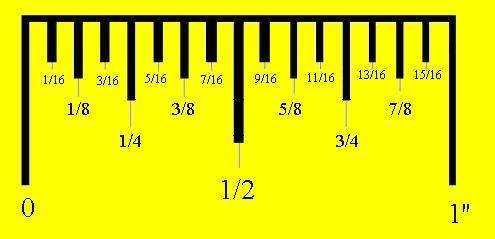# How do I measure with a tape measure?

Below is an article on how to read a tape measure. The original article can be read by clicking here.Above is what you’ll find at the beginning of an imperial tape measure. Each inch is broken down into sixteen segments. In order to read and understand an imperial tape measure, you will need to have a basic understanding of fractions.
Although every segment is 1/16th of an inch, you don’t read the halfway point as 8/16ths, because you can ‘simplify’ that fraction. If both numbers can be divided by one number then thats what you do in order to simplify. Both 8 and 16 can be divided by 8, so 8/16 is also 1/2 inch.
Here is each increment of one inch, simplified;
1/16
2/16 = 1/8
3/16
4/16 = 2/8 = 1/4
5/16
6/16 = 3/8
7/16
8/16 = 4/8 = 2/4 = 1/2
9/16
10/16 = 5/8
11/16
12/16 = 6/8 = 3/4
13/16
14/16 = 7/8
15/16
16/16 = 1
It’s confusing at first but becomes second nature after a short while. It does get a little more complicated if you have to add two measurements together. This is fairly straight forward if the bottom numbers of the fraction are the same,
1/4 + 1/4 = 2/4 or 1/2
And
2/4 + 3/4 = 5/4 or 1 and a 1/4
If the bottom numbers are different then it takes a little more work;
1/16 + 1/8
Here, you need to make the numbers under the lines the same. Change 1/8 back to 2/16 so they can be added together;
1/16 + 2/16 = 3/16
Another example;
3/8 + 15/16
Change 3/8 to 6/16 to make it work
6/16 + 15/16 = 21/16 0r 1 and 5/16